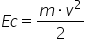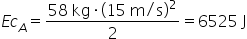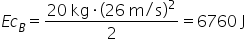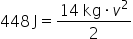Definitions

# Kinetic Energy

Kinetic energy is a form of energy that is produced when an object, substance, or particle is in motion . This energy depends mainly on the speed of the object, in addition to its mass . For example, when we run, our body is in motion, so it produces kinetic energy.

It is usually abbreviated with the letters “Ec” or “Ek”, and can be expressed with the following formula:Below we explain each term and the units used according to the International System:

• Ec : is the kinetic energy measured in Joules or Joules, J .
• m : is the mass of the moving body, measured in kilograms, kg .
• v : is the speed of the body, measured in meters per second, m/s . Since this term is squared ( 2 ), the final units are 2 /s 2 .
• The equation is divided by 2 , since the kinetic energy formula is the result of deriving and integrating the Newton’s Second Law formula (Force = Mass · Acceleration) into the work formula (Work = Force · Displacement).

It is linked to other concepts in physics such as work and force. The object, being in motion, causes a determined work and force. For example, if it causes enough force, the object will be able to deform or displace another.

A moving object can return to its initial state or reach a state of rest if another force acts on it. The work caused by the external force counteracts that of the kinetic energy of the object. For example, a wheel going down a slope until it hits a wall. The wall applies a greater force on the wheel, so the wheel will stop moving, reducing its kinetic energy to zero.

Likewise, an object generates kinetic and/or potential energy. When the object is at rest, it is zero, while the potential energy is maximum. If the object is in motion and there is no other force acting on it, it generates kinetic energy, but not potential energy. Finally, an object moving within a gravitational field always generates kinetic and potential energy.

## Types and examples

There are three types: translation, rotation, and vibration.

Translational occurs when an object moves from one point to another. When moving in one direction, the acceleration applied to the mass of the object is translated into kinetic energy. Some examples are the action of walking, driving a vehicle on the highway or falling objects.

Rotational occurs when an object rotates on itself. Although there is no displacement, part of the object is accelerating around an axis, generating kinetic energy. Examples of this are the movement of the discs in players, the yo-yo and the movement of the blades of a windmill due to the wind.

The kinetic energy of vibration occurs when the particles oscillate around a point of equilibrium. Some examples are the vibration of the mobile, the movement of the strings of a guitar or the molecular vibration.

Also, kinetic energy can be represented in other types depending on the effect or type of energy they generate. These are:

• Mechanical energy : the movement of objects and their physical interaction with others produces a mechanical movement, the result of kinetic and potential energy. An example of this is when we hit a ball. The kinetic energy of our foot is transferred to the ball, producing mechanical energy in the form of displacement.
• Thermal energy : the vibration and movement of the particles of a substance gives off heat, that is, thermal energy. Depending on the state of aggregation of matter (solid, liquid or gas), the thermal energy will be higher or lower.
• Electrical energy : Electrons, when moving through an electrical circuit, generate electricity from their kinetic and potential energy.
• Sound energy : It is a type of mechanical energy that results from the oscillation or vibration of particles in the form of waves. This oscillation generates sound waves that the ear can pick up.

## Examples of exercises using the kinetic energy formula

### Exercise 1

An object A of mass 58 kilograms is moving at 15 meters per second, while an object B of mass 20 kilograms is moving at 26 meters per second. Which of the two objects generates more kinetic energy?

To find out, let’s first calculate the kinetic energy of object A:Next, let’s calculate the kinetic energy of object B:By comparing both figures, we can conclude that object B generates more kinetic energy than object A. The speed of an object, when squared in the formula, has a greater impact on kinetic energy than mass.

### Exercise 2

Imagine that a bowler throws a ball of mass 14 kilograms. When the ball collides with the pins or pins, a kinetic energy of 448 Joules is detected. How fast was the ball traveling before it hit?

If we substitute the terms of the equation, we have:If we clear the speed in the formula, we have:Therefore, the ball was moving at 8 meters per second before colliding with the pins. Remember that 1 Joule is equal to 1 (kg·m 2 )/s 2 .